CATEGORIES:

# Inverse Matrices

Definition. A square matrix is said to be nondegenerate, if it has nonzero determinant.

Consider the identity matrix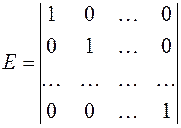.

Definition. The inverse matrix for А is the matrix А–1for whichили. (11)

Inverse matrices exist only for nondegenerate matrices.

The first method for finding the inverse matrix. Consider a square nondegenerate matrix of size nn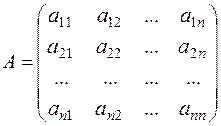.

Let us compose the square matrix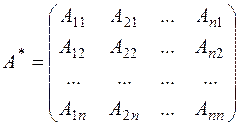,

where the Аij are the algebraic complements of аij.

The matrix А* is called the adjoint matrix.

Let us multiply А by А* :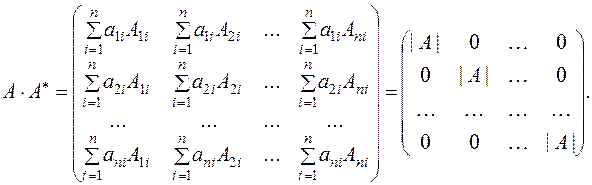The remaining elements of the first row in the new matrix are the sum of the products of the elements of the first row and the algebraic complements of the parallel rows. According to property 10, they equal zero. The same is true for the elements of the second row, except the second element, which are the sum of the products of the elements of the second row by the algebraic complements of the first, third , ... rows.

To obtain the identity matrix from this diagonal matrix, we must divide it by the determinant, i.e.,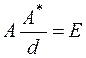;

consequently,,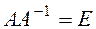,. (12)

Example. Find the inverse matrix for the matrix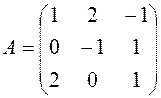,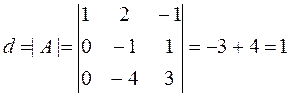,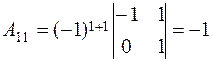,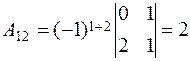,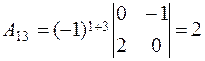,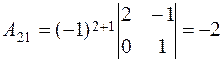,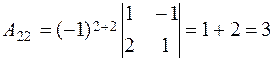,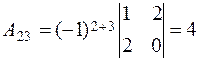,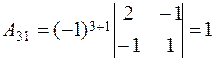,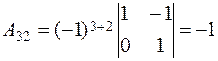,,.

Let us verify condition (11) from the definition of the inverse matrix:.

The second method for finding the inverse matrix. This method is similar to the Gauss-Jordan method. Applying the Jordan transformations to the rows of the principal matrix, we reduce it to the identity matrix, and the identity matrix added to it becomes the inverse matrix.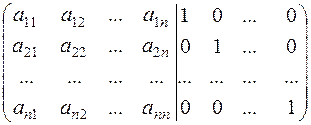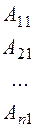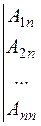Example.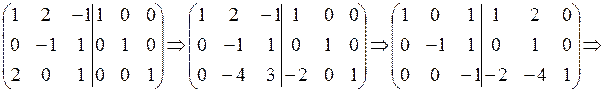.

Matrix Method for Solving Systems of Equations

Consider a system of n equations with n unknowns: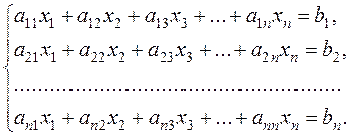(13)

Let us find a solution of system (13), by using matrices.

The matrix method applies only where the number of equations equals that of unknowns. Let us write system (13) in matrix form; for this purpose we introduce, principal matrix А, the column matrix Х, and the column matrix of free terms В: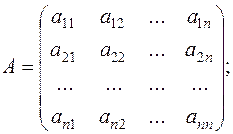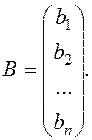Then system (13) can be written in the form of the matrix equation

АХ=В.

Two matrices of the same size are equal if and only if each element of one matrix equals the corresponding element of the other matrix. To find the matrix Х, we multiply both sides of the matrix equation by the inverse matrix А-1 on the left.

Sinceis the identity matrix, we have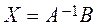. (14)

Thus, to solve the given system of equations by the matrix method, it is sufficient to find the inverse matrix А-1 and multiply it by В on the right.

Example. Solve the following system of equations by the matrix method:Let us write the system in matrix form:.

Applying (14), we obtain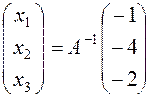.

The principal matrix is nondegenerate, and its determinant equals d=6.

Now, let us find the inverse matrix: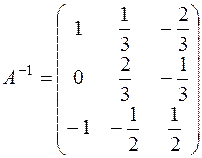.

The solution of the system is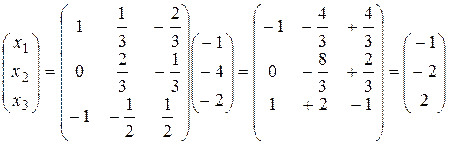.

Thus, the solution of the system is x1= –1, x2= –2, x3=2.

Date: 2015-01-02; view: 988

 <== previous page | next page ==> Rank of a Matrix | LECTURE 3.
doclecture.net - lectures - 2014-2021 year. Copyright infringement or personal data (0.003 sec.)